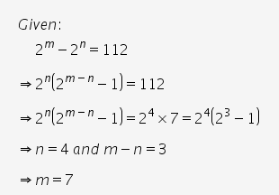# Two finite sets have m and n elements. The total number of subsets of the first set

Two finite sets have m and n elements. The total number of subsets of the first set is 112 more than the total number of subsets of the second set. Find the values of m and n.